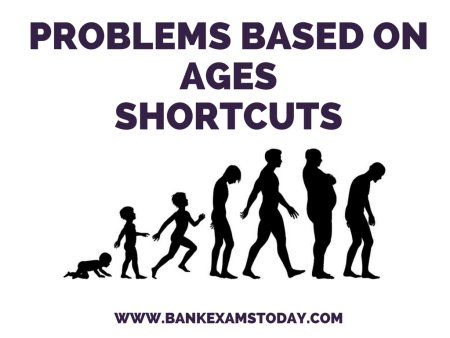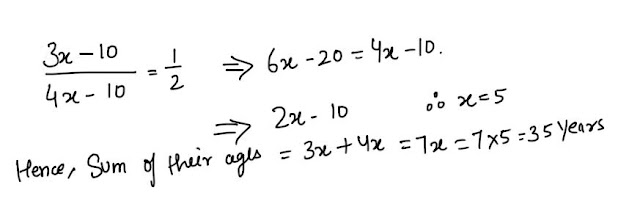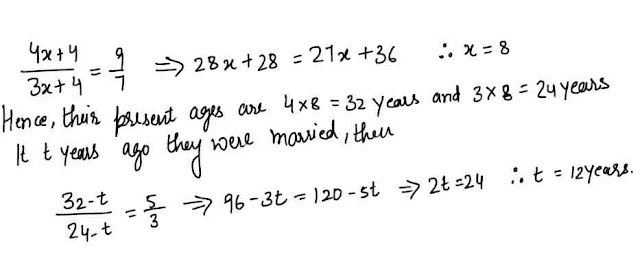# Problem Based on Ages - 12 Repeated Questions in the Exams#### Ques 1.

The sum of present ages of Ashok, Anil and Ajay is 75 years.If 5 years ago the ratio of their ages was 3 : 4 : 5, What is the present age of Anil?
Ans 1. Let 5 years age, the age of ashok, Anil and Ajay were 3x, 4x and 5x years. Then, their present ages are ( 3x + 5), (4x + 5)  abd ( 5x + 5) years.
Now, 3x +5 + 4x +5 + 5x + 5 = 75   =>  12x   = 60       x = 5
Hence, present age of Anil  =  4x + 5  = 4 x 5 + 5 = 25 years.

#### Ques 2.

The ratio of ages of Ajay and vijay at present is 3 :2 :8 years earlier, the ratio was 5 : 3. What are their present ages?
Ans 2.  Let the present ages of Ajay and Vijay  are 3x years  and 2x years respectively ; then#### Ques 3.

One year ago the ratio between samir and Saurabh's age was 4 : 3 One year hence, the ratio of their ages will be 5 : 4 .What is the sum of their present ages in years ?
Ans 3. Let one year ago ages of Samir and Saurabh was 4x and  3x  years respectively; then#### Ques 4.

The average age of a man and his son is 48 years. The ratio of their age is 5 : 3 respectively .What is the son's age ?
Ans 4. Sum of ages of man and his son = 2 x 48 = 96
Let the ages of man and his son be  5x and 3x years; then
5x + 3x  = 96               =>  x = 12
Hence, son's age  = 3x = 3 x12 = 36 years.

#### Ques 5.

The ratio of ages of Namrata and Divya is 4 : 3 .The sum of their ages is 28 years.The ratio of their ages after 4 years will be :
(a) 3: 4
(b) 5 : 4
(c) 5 : 6
(d) 6 :5
Ans 5.  Let the present ages of Namrata and Divya are 4x and 3x years  respectively;then  4x + 3x = 28
Hence, their present ages are 16 and 12 years.
so, required ratio = ( 16 + 4) : ( 12 + 4) = 20 : 16  = 5 : 4

#### Ques 6.

Ten years ago, the age of Divya was half of the age os namrata .If the ratio of present ages of both is 3 :4 the sum of their present ages is :
(a) 35 years
(b) 30 years
(c) 25 years
(d) 18 years
Ans 6. Let the present ages of Divya and Namrata are 3x and 4x years respectively ; then#### Ques 7.

The ratio of ages of a man and his wife is 4:3 .After 4 years, this ratio will be 9:7 . If at time of marriage, the ratio was 5 :3, then how many years ago were they married ?
(a) 15 years
(b) 12 years
(c) 10 years
(d) 8 years
Ans 7. Let the present ages of the man and his wife are 4x and 3x years respectively ; then#### Ques 8.

Sudha's grandfather was 8 times older to her 16 years ago.He would be 3 times of her age 8 years from now. Eight years ago, What was the ratio of Sudha's age to that to her grandfather?
(a)11:53
(b) 3: 8
(c) 1 : 5
(d) 1: 2
Ans 8. Let 16 years ago, the age of Sudha and her grandfather were x and 8x years respectively; then#### Ques 9.

The sum of ages of A and B is 12 years more than the sum of ages B and C.Then C is how many years younger than A ?
(a) 10
(b) 12
(c) 24
Ans 9. Here, ( A+B) - ( B + C) = 12      A -C = 12
Hence,C is 12 years younger than A.

#### Ques 10.

The sum of the ages of 5 children born at the interval of 3 years each is 50 years.the age of youngest child is :
(a) 10 years
(b) 8 years
(c) 4 years
(d) None of these
Ans 10. Let the age youngest child be x  years ; then
x +  ( x + 3) + x + 6) + x+ 9) + ( x + 12) = 50        => 5x = 20                  . x = 4 years

#### Ques 11.

A father said to his son,"I was as old as you are at present at the time of your birth." If the father's age is 38 years now. Five years ago the age of son was:
(a) 38 years
(b) 33 years
(c) 19 years
(d) 14 years
Ans 11. Let the age of father was x years at the time of his son's birth then present age of father and his son will be 2x and x years.
Now, 2x = 38       .  x = 19 years
Hence, 5 years ago the age of son was 19 -5 = 14 years.

#### Ques 12.

Nisha's father was 38 years of age when she was born while her mother was 36 years old when her brother four years younger to her was born. The difference of ages of her parents is :
(a) 8 years
(b) 6 years
(c) 4 years
(d) 2 years
Ans 12. The difference of ages of her parents = ( 38 + 4) - 36 = 43 -36  = 6 years.#### What's trending in BankExamsToday

Smart Prep Kit for Banking Exams by Ramandeep Singh - Download here